# Lecture 21 Options Markets Options With options one

• Slides: 31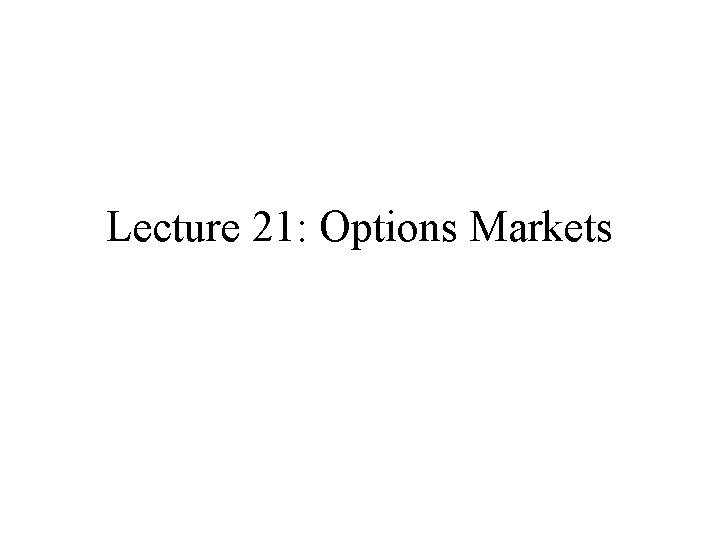Lecture 21: Options Markets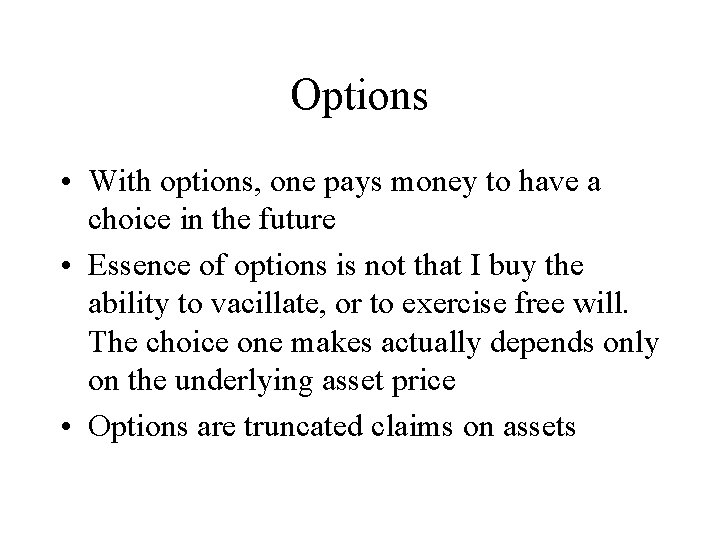Options • With options, one pays money to have a choice in the future • Essence of options is not that I buy the ability to vacillate, or to exercise free will. The choice one makes actually depends only on the underlying asset price • Options are truncated claims on assets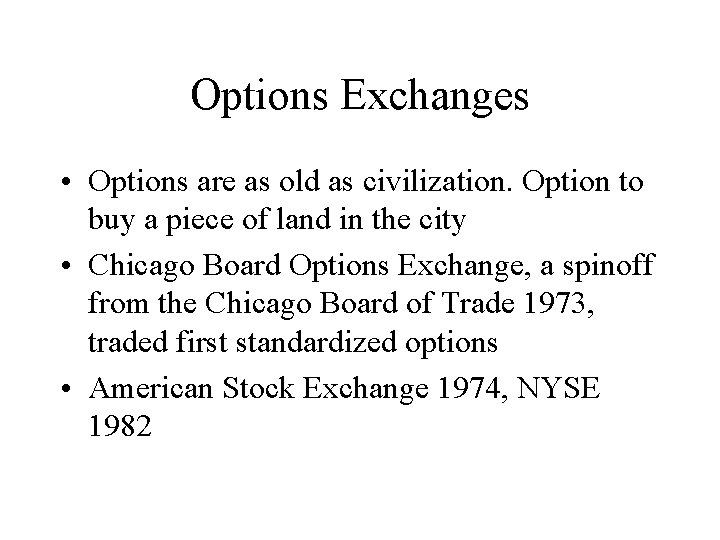Options Exchanges • Options are as old as civilization. Option to buy a piece of land in the city • Chicago Board Options Exchange, a spinoff from the Chicago Board of Trade 1973, traded first standardized options • American Stock Exchange 1974, NYSE 1982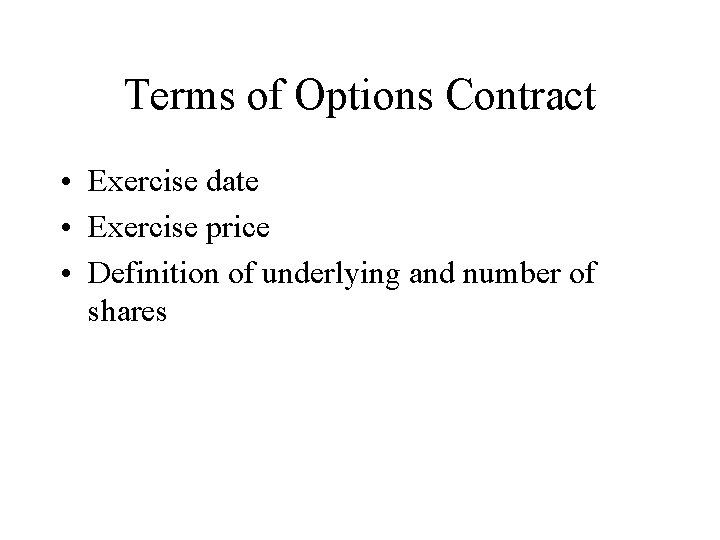Terms of Options Contract • Exercise date • Exercise price • Definition of underlying and number of shares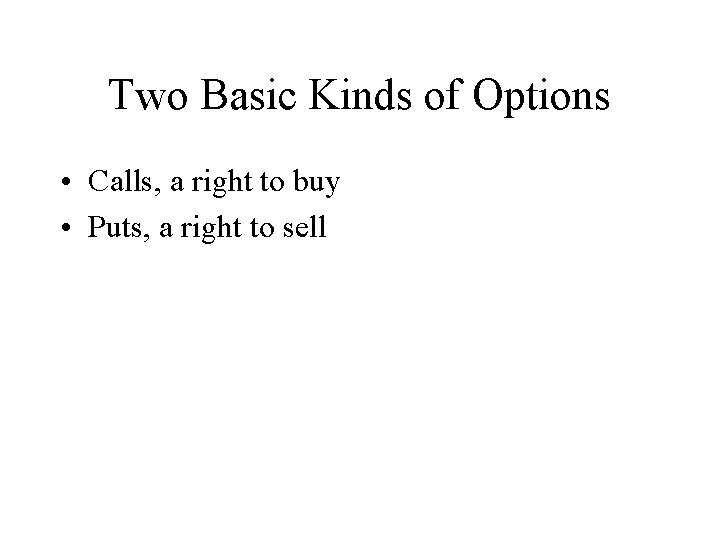Two Basic Kinds of Options • Calls, a right to buy • Puts, a right to sell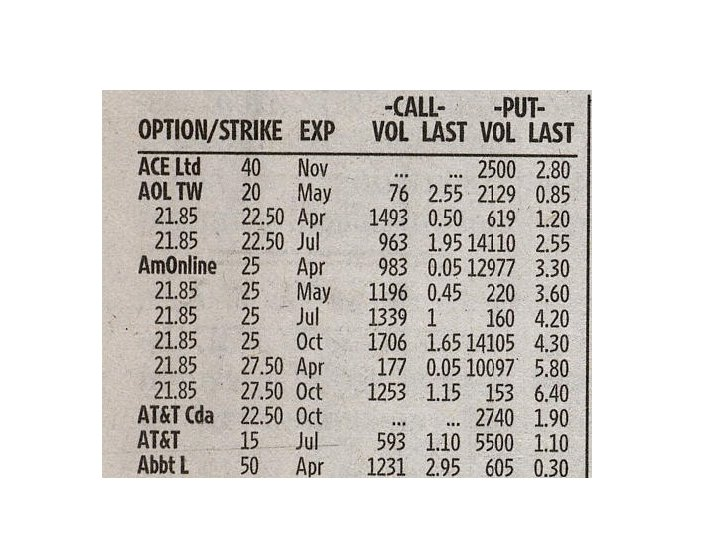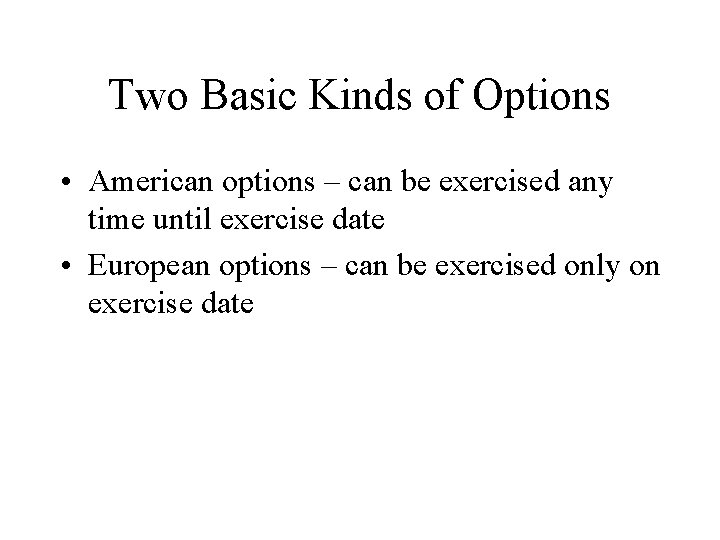Two Basic Kinds of Options • American options – can be exercised any time until exercise date • European options – can be exercised only on exercise dateBuyers and Writers • For every option there is both a buyer and a writer • The buyer pays the writer for the ability to choose when to exercise, the writer must abide by buyer’s choice • Buyer puts up no margin, naked writer must post margin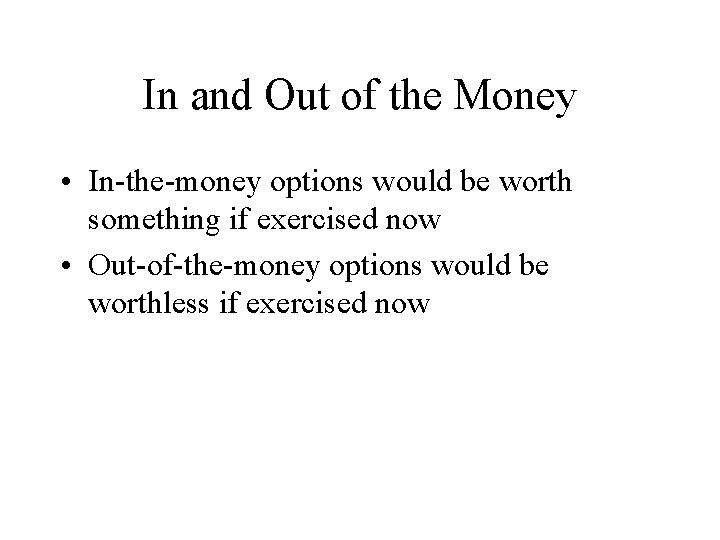In and Out of the Money • In-the-money options would be worth something if exercised now • Out-of-the-money options would be worthless if exercised nowPut-Call Parity Relation • Put option price – call option price = present value of strike price + present value of dividends – price of stock • For European options, this formula must hold (up to small deviations due to transactions costs), otherwise there would be arbitrage profit opportunitiesLimits on Option Prices • Call should be worth more than intrinsic value when out of the money • Call should be worth more than intrinsic value when in the money • Call should never be worth more than the stock priceBinomial Option Pricing • Simple up-down case illustrates fundamental issues in option pricing • Two periods, two possible outcomes only • Shows how option price can be derived from no-arbitrage-profits condition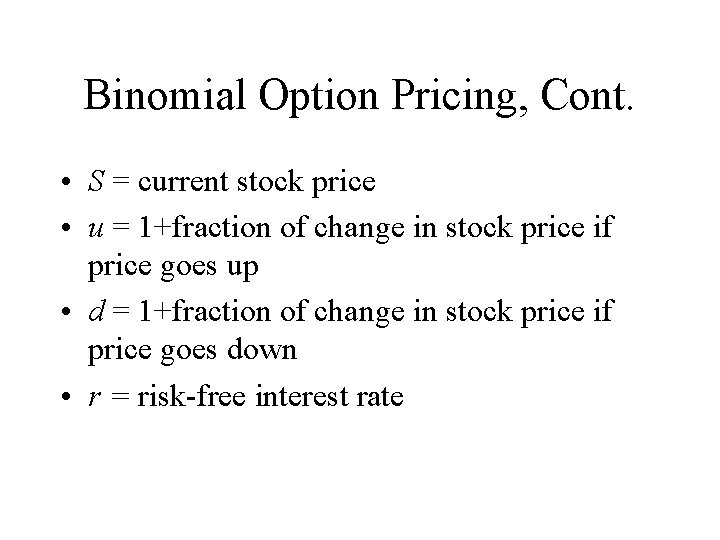Binomial Option Pricing, Cont. • S = current stock price • u = 1+fraction of change in stock price if price goes up • d = 1+fraction of change in stock price if price goes down • r = risk-free interest rateBinomial Option Pricing, Cont. • C = current price of call option • Cu= value of call next period if price is up • Cd= value of call next period if price is down • E = strike price of option • H = hedge ratio, number of shares purchased per call sold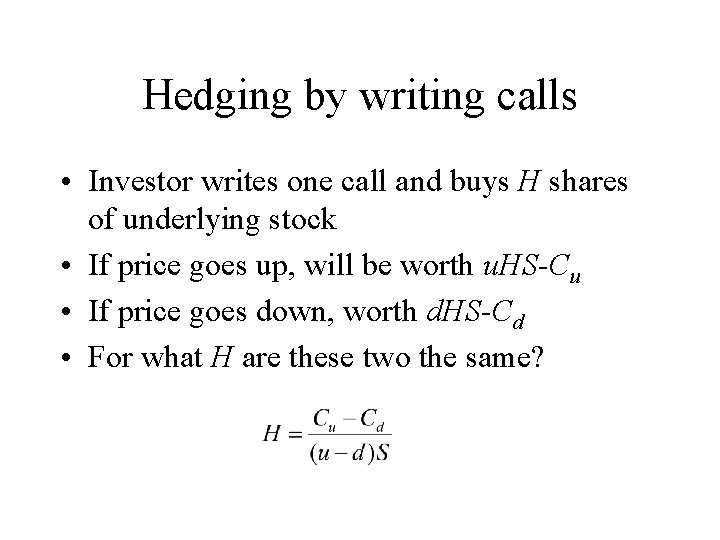Hedging by writing calls • Investor writes one call and buys H shares of underlying stock • If price goes up, will be worth u. HS-Cu • If price goes down, worth d. HS-Cd • For what H are these two the same?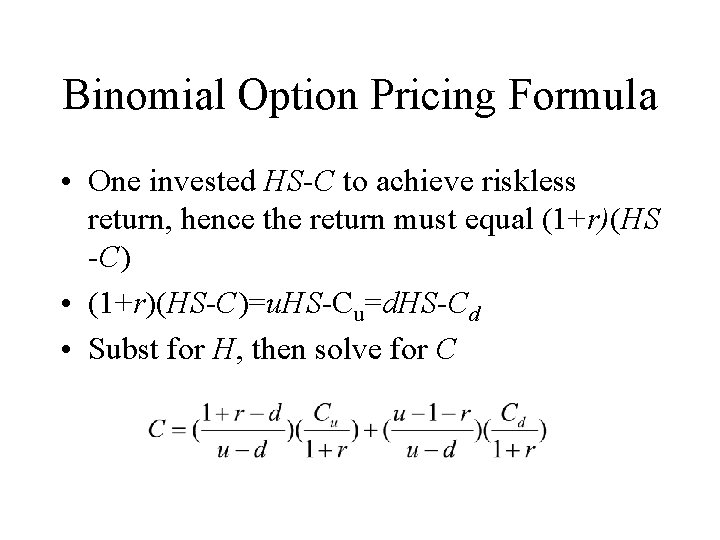Binomial Option Pricing Formula • One invested HS-C to achieve riskless return, hence the return must equal (1+r)(HS -C) • (1+r)(HS-C)=u. HS-Cu=d. HS-Cd • Subst for H, then solve for C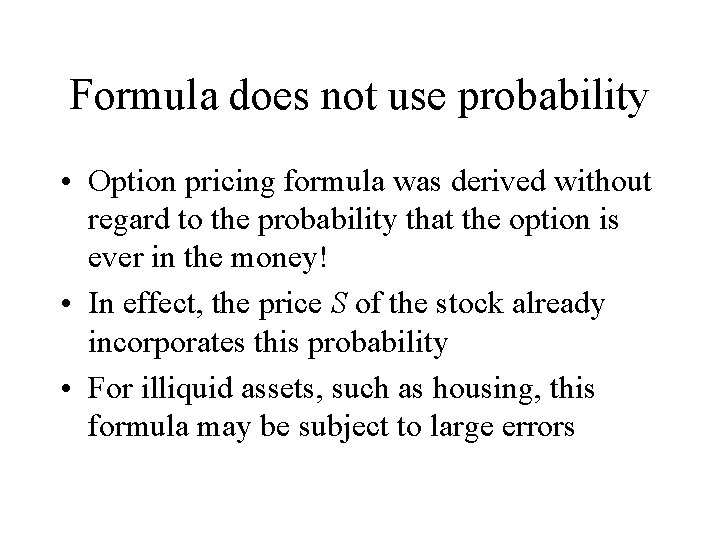Formula does not use probability • Option pricing formula was derived without regard to the probability that the option is ever in the money! • In effect, the price S of the stock already incorporates this probability • For illiquid assets, such as housing, this formula may be subject to large errorsBlack-Scholes Option Pricing • Fischer Black and Myron Scholes derived continuous time analogue of binomial formula, continuous trading, for European options only • Black-Scholes continuous arbitrage is not really possible, transactions costs, a theoretical exercise • Call T the time to exercise, σ2 the variance of oneperiod price change (as fraction) and N(x) the standard cumulative normal distribution function (sigmoid curve, integral of normal bell-shaped curve) =normdist(x, 0, 1, 1) Excel (x, mean, standard_dev, 0 for density, 1 for cum. )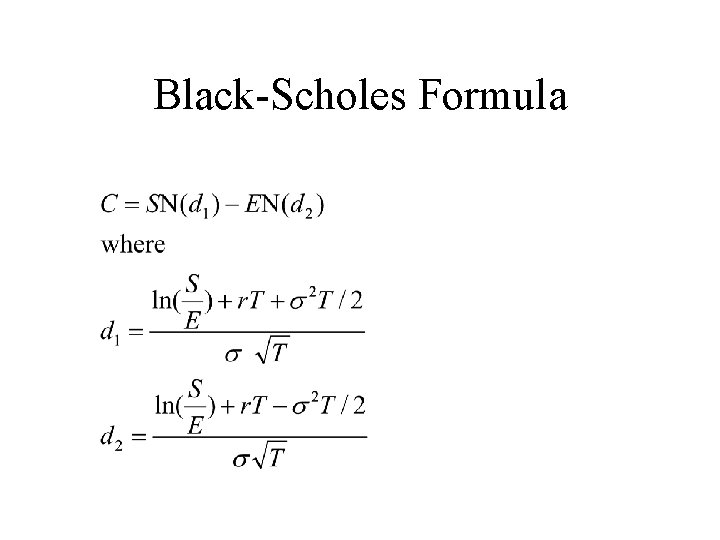Black-Scholes Formula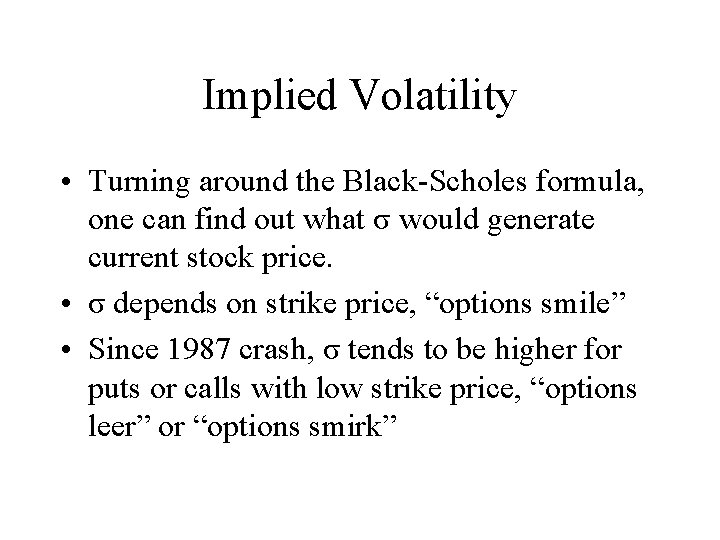Implied Volatility • Turning around the Black-Scholes formula, one can find out what σ would generate current stock price. • σ depends on strike price, “options smile” • Since 1987 crash, σ tends to be higher for puts or calls with low strike price, “options leer” or “options smirk”VIX Implied Volatility Weekly, 1992 -2004Implied and Actual Volatility Monthly Jan 1992 -Jan 2004Actual S&P 500 Volatility Monthly 1871 -2004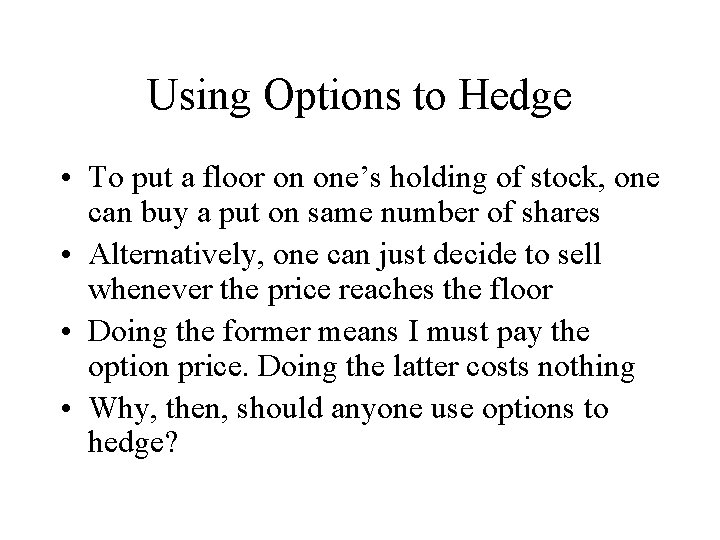Using Options to Hedge • To put a floor on one’s holding of stock, one can buy a put on same number of shares • Alternatively, one can just decide to sell whenever the price reaches the floor • Doing the former means I must pay the option price. Doing the latter costs nothing • Why, then, should anyone use options to hedge?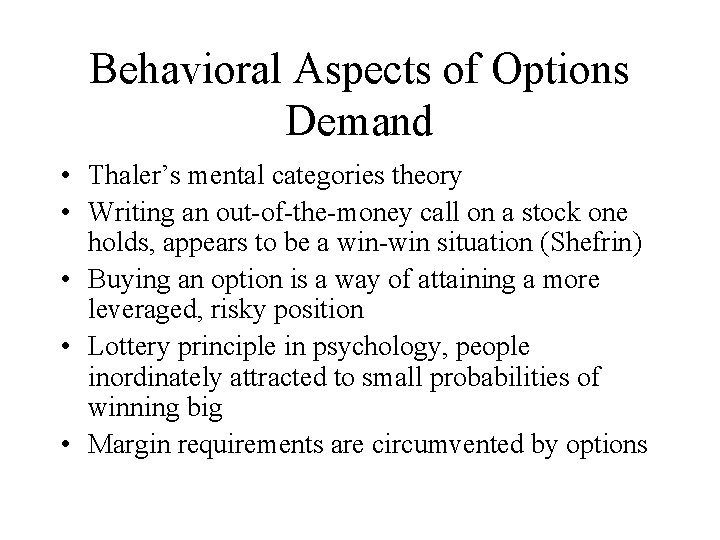Behavioral Aspects of Options Demand • Thaler’s mental categories theory • Writing an out-of-the-money call on a stock one holds, appears to be a win-win situation (Shefrin) • Buying an option is a way of attaining a more leveraged, risky position • Lottery principle in psychology, people inordinately attracted to small probabilities of winning big • Margin requirements are circumvented by optionsOption Delta • Option delta is derivative of option price with respect to stock price • For calls, if stock price is way below exercise price, delta is nearly zero • For calls, if option is at the money, delta is roughly a half, but price of option may be way below half the price of the stock. • For calls, if stock price is way above the exercise price, delta is nearly one and one pays approximately stock price minus pdv of exercise price, like buying stock with credit pdv(E)